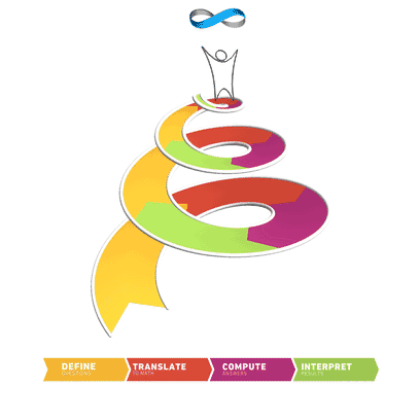There are some great ways to link computing and all the other subjects on the school curriculum, but there’s a particularly strong argument for making connections with mathematics.

As a discipline, computing owes its origins to mathematics. Babbage’s analytical engine was designed to compute mathematical tables. The word ‘computer’ was originally applied to a person who performed calculations. Turing’s paper ‘On computable numbers with an application to the Entscheidungsproblem’ was more about proving a mathematical theorem than founding a new science. Cambridge’s computer lab started out as ‘the mathematical laboratory‘.

Let’s look at the connections from both perspectives: firstly, bringing maths into computing.

Given our current focus on thinking rather than coding, there are strong parallels between mathematical reasoning and computational thinking: logical reasoning, algorithms, abstraction, generalisation, decomposition and evaluation play a central role in both CS and mathematical problem solving. Conrad Wolfram’s four steps for doing maths parallel stages of traditional software development:

1. define
2. translate
3. compute
4. interpretCBM Solution Helix of Math

There’s a fair amount of mathematical content in computer science as taught in schools. In primary, Bee Bots and similar are used early on to practise positional language and emerging measure and children are now more likely to meet angles and coordinates in Scratch before these get covered in maths lessons. Topics such as binary conversion, binary arithmetic, Boolean logic and functions all make demands on pupils’ mathematical understanding. There’s some potentially gritty maths in computing at GCSE and A level: graph theory, modular arithmetic, formal logic and measures of algorithmic efficiency.  It’s perhaps no surprise that grade B in GCSE maths is seen as a prerequisite for A Level computer science, or that A level maths / further maths, is often a requirement for studying CS at Russell Group universities.

Going in the other direction, there are very many opportunities to enrich school mathematics through computing, but more than that, computer science provides a means through which mathematical ideas become “incarnate, tangible and executable”.

Wolfram makes a strong case for ‘computer based mathematics’ being taught in schools – arguing that this reflects how real maths is done, making the subject far more relevant than when calculations are done by hand. It’s the third of Conrad’s steps (‘compute’) that the machines are unsurprisingly much better at: perhaps a pupil’s mathematical understanding would be enhanced by spending more of her time on steps 1, 2 and 4 and proportionately less on the easily automatable step 3. ‘Show your working’ could be at least as much about providing the source code for your program as making marks on paper.

In much of maths education working with software can be powerful: turtle graphics as a tool to think geometrically; spreadsheets as a visual introduction to functional programming; the classes, objects and methods of dynamic geometry software; data visualisation without the faff through Excel, Raw or R.

There’s a place for introducing pupils to the tools (some) professionals use, such as Mathematica or its slimmed down, online sibling, Wolfram Alpha. Mathematica is programmed using the Wolfram Language, which is very high level, with built-in functions for finite state machines, graph traversals, Turing machines and machine learning classifiers, amongst other things… Note Mathematica is bundled as part of NOOBS for the Raspberry Pi.

Programming has many applications in maths education: writing functions for fractions arithmetic, solving equations numerically, simulating probability experiments etc. The list is pretty inexhaustible, and provides rich territory to develop understanding in mathematics and computer science side by side.

The links between computer science and mathematics provide both challenge and opportunity for curriculum development in schools. We draw on mathematics in computing; we use computation (automated or otherwise) in mathematics. At the recent Computer Based Mathematics summit, CAS chair Simon Peyton Jones and Andrew Fitzgibbon made a strong case for teaching the two subjects together – with the current curricula and exam specs for the two subjects. What they suggest isn’t maths with a nod to CS or CS with a nod to maths, but rather CS bringing to life the abstractions of maths, and maths providing the foundational abstractions that underpin CS. Implementing such a vision at national scale isn’t trivial, but perhaps a few teachers in some forward thinking schools or academies might be up for trying this out? It would be phenomenally interesting experiment!

Originally published in the Spring 2016 edition of Switched On, the Computing at School newsletter, as an introduction to a section on computing and mathematics.